# How To Find The Perimeter Of A Square (3 Things To Know)

The perimeter of a square tells us how far we would have to walk to go around a square, walking along each side and turning 90 degrees at the corners.  It is useful to know how to find the perimeter of a square given information about the square.

So, how do you find the perimeter of a square?  A square with a side length of S has a perimeter of 4S (this comes from adding up all four sides, each of length S). Side length and perimeter are always given in linear units (such as feet or inches). To find the side length S of a square with perimeter P, we divide P by 4 to get S = P/4.

Of course, we can also find the perimeter of a square given its area, diagonal, or other information.

In this article, we’ll talk about how to find the perimeter of a square and look at some examples.  We’ll also answer some common questions about perimeters of squares.

Let’s get started.

## How To Find The Perimeter Of A Square

To find the perimeter of a square, we need to know the side length, or find it from the information we are given.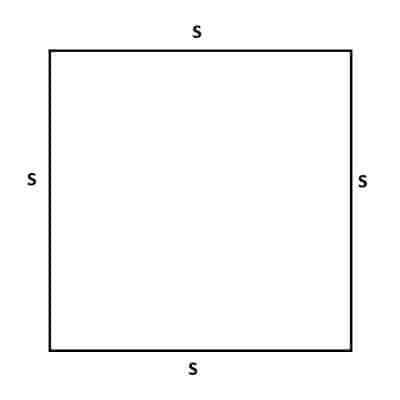If we know the side length S of a square (or if we can find it from information we are given), then we can find the perimeter P.

Remember: a square has four sides of equal length and four 90 degree angles.  This means that we only need to have one side length of a square and we know all the side lengths.

Let’s start off with the simplest case: finding the perimeter of a square given its side length.

DIY Shed AsktheBuilder Shed Foundat...
DIY Shed AsktheBuilder Shed Foundation Layout

### How To Find The Perimeter Of A Square With Side Length

If we have the side length of a square, it is easy to find the perimeter.  For a square with a side length of S, the perimeter P is given by the formula:

• P = 4S  [formula for the perimeter of a square]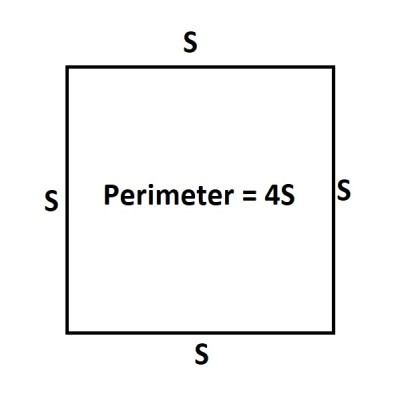A square with a side length of S has a perimeter of 4S.

Note that S (side length of the square) and P (perimeter of the square) are given in linear units, such as:

• Feet (ft)
• Inches (in)
• Meters (m)

Let’s look at an example of how to find the perimeter of a square, given the side length.

#### Example: Find The Perimeter Of A Square Given The Side Length

Let’s say we have a square with a side length of 5 feet.

Then S = 5 feet, and we can use this in the formula for the perimeter P of a square:

• P = 4S  [formula for the perimeter of a square]
• P = 4(5) [units for S are feet]
• P = 20 feet

So, the perimeter of a square with a side length of 5 feet is 20 feet.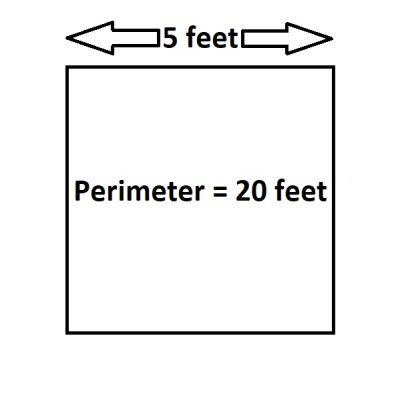A square with a side length of 5 feet has a perimeter of 20 feet (4*5 = 20).

### How To Find The Perimeter Of A Square With The Area

Remember that a square with a side length of S has an area of A = S2.  This comes from multiplying the width by the height (each has the same length of S):

• A = S*S = S2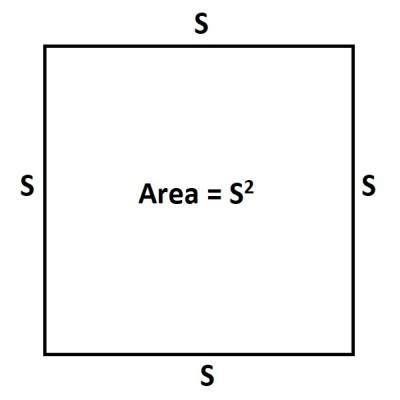A square with a side length of S has an area of S2.

We can also work backwards to find the side length S from the area A.  All we need to do is take a square root:

• A = S2
• √A = S

Since we know the perimeter P in terms of S, we can find the perimeter in terms of the area too:

• P = 4S  [formula for the perimeter of a square]
• P = 4(√A)  [solved for side length, S, in terms of area, A]
• P = 4√A

The previous line is the formula for the perimeter P of a square with area A.

Note that we can also work backwards to find the area A from the perimeter P by solving:

• P = 4√A  [formula for perimeter P in terms of area A]
• P2 = 16A
• P2/16 = A

Let’s look at an example of how to find the perimeter of a square given its area.

#### Example: Find The Perimeter Of A Square With The Area

Let’s say we have a square with an area of 36 square feet.

Then A = 36 square feet, and we can use this in the formula for the perimeter P of a square that we found above:

• P = 4√A  [formula for perimeter P in terms of area A]
• P = 4√(36)  [units for A are feet]
• P = 4*6 feet
• P = 24 feet

So, the perimeter of a square with an area of 36 square feet is 24 feet.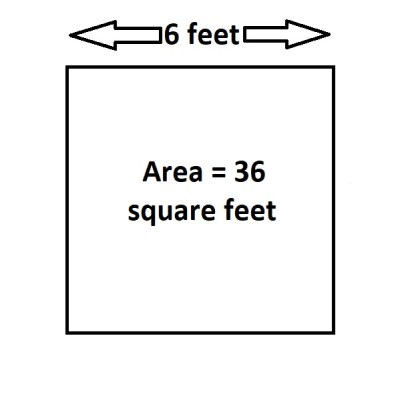A square with an area of 36 square feet has a side length of 6 feet, and thus a perimeter of 4*6 = 24 feet.

We can also check our work by finding the side length S.  For a square with an area of 36 feet, the side length will be S = √A = √36 = 6 feet.

A square with a side length of S = 6 feet will have a perimeter of P = 4S = 4(6) = 24 feet.  This matches what we found above.

### How To Find The Perimeter Of A Square With The Diagonal

Remember that a square with a side length of S has a diagonal of D = S√2.  This comes from using the Pythagorean Theorem on one of the right triangles (half of the square, with the diagonal as the hypotenuse):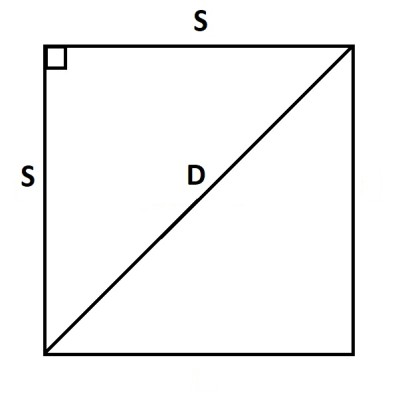A square with a diagonal D and side length S satisfies the equation D = S√2.
• a2 + b2 = c2  [Pythagorean Theorem]
• S2 + S2 = D2  [legs a and b are the side length S of the square, and c is the diagonal D]
• 2S2 = D2  [combine like terms]
• √(2S2) = D  [take square root of both sides]
• S√2 = D  [simplify]

This also means that we can solve for the side length S to find:

• S√2 = D
• S = D/√2

Since we know the perimeter P in terms of S, we can find the perimeter in terms of the diagonal too:

• P = 4S  [formula for the perimeter of a square]
• P = 4(D/√2)  [solved for side length, S, in terms of diagonal, D]
• P = 4√2(D/2)  [rationalize the denominator]
• P = (2√2)D

The previous line is the formula for the perimeter P of a square with diagonal D.

Note that we can also work backwards to find the diagonal D from the perimeter P by solving:

• P = (2√2)D  [formula for perimeter P in terms of diagonal D]
• P/(2√2) = D
• √2P/(2*2) = D  [rationalize the denominator]
• √2P/4 = D

Let’s look at an example of how to find the perimeter of a square given its diagonal.

#### Example: Find The Perimeter Of A Square Given The Diagonal

Let’s say we have a square with a diagonal of 10 feet.

Then D = 10 feet, and we can use this in the formula for the perimeter P of a square that we found above:

• P = (2√2)D  [formula for perimeter P in terms of diagonal D]
• P = (2√2)(10)  [units for D are feet]
• P = 20√2 feet

So, the perimeter of a square with a diagonal of 10 feet is 20√2 feet (about 28 feet).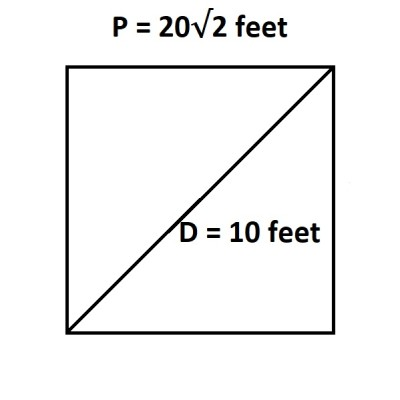A square with a diagonal 10 feet long has a perimeter of 20√2 feet.

We can also check our work by finding the side length S.  For a square with a diagonal of 10 feet, the side length will be S = D/√2 = 10/√2 = (10√2)/2 = 5√2 feet.

A square with a side length of S = 5√2 feet will have a perimeter of P = 4S= 4(5√2) = 20√2  feet.  This matches what we found above.

### How To Find The Perimeter Of A Square Inscribed In A Circle

When you inscribe a square inside of a circle, you are really looking the biggest square that will just barely fit inside of the circle.  You can also think of it as the smallest possible circle that just barely contains the square.

This scenario is illustrated in the image below.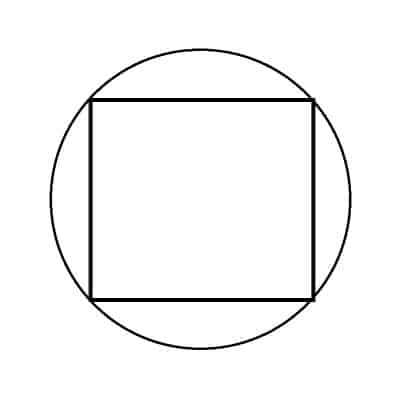Here we see the largest square that can fit inside the circle (or the smallest circle that can contain the square).

We can find the relationship between these two shapes and their dimensions as follows.

Let S be the side length of the square, and let R be the radius of the circle.

First, we will draw a diameter from the bottom left corner of the square to the top left corner.  This gives us a diagonal with length R + R = 2R (the diameter of the circle).

We will label the sides of the square “S” (as shown in the diagram below).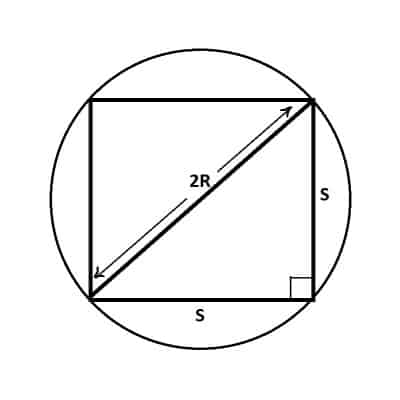The diagonal of the square is twice the radius (diameter) of the circle, or 2R. The side lengths are also labeled on the right triangle.

At this point, we have a right triangle whose two short sides (legs) have lengths of S and S, with a hypotenuse of 2R (the circle’s diameter).  Using the Pythagorean Theorem, we get:

• a2 + b2 = c2  [a and b are the side lengths, and c is the hypotenuse]
• S2 + S2 = (2R)2  [the side lengths are both S, and the hypotenuse or diagonal is 2R, the circle’s diameter]
• 2S2 = 4R2  [combine like terms]
• S2 = 2R2  [divide by 2]
• S = R√2  [take the square root of both sides]

This equation gives us the connection between the radius R of the circle and the side length S of the square.

This tells us that the side length S of the square will be about 41% larger than the radius of the circle (√2 is about 1.41 as a decimal).

The perimeter of the square is P = 4S = 4(R√2) = (4√2)R.

Let’s look at an example.

#### Example: How To Find The Perimeter Of A Square With A Circle Inscribed

Let’s say that we have a square inscribed inside a circle, as above.  The circle has a diameter of 12 feet.

This means that the radius (half the diameter) is 12/2 = 6 feet.  So, R = 6 feet.

We can now use the formula we found above for the perimeter P of the square inscribed in the circle:

• P = (4√2)R  [area of the square inscribed in the circle of radius R]
• P = (4√2)(6)  [R = 6 feet]
• P = 24√2 feet
• P ~ 34 feet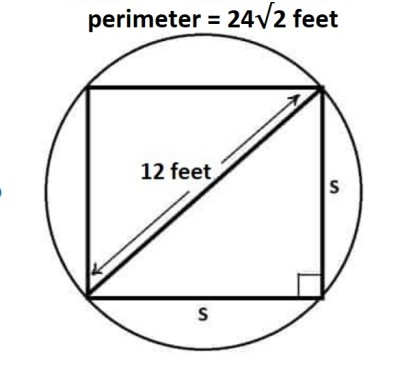A square inscribed in a circle of diameter 12 feet has a perimeter of 24√2 square feet.

### How To Find The Side Length Of A Square When Perimeter Is Given

To find the side length of a square when the perimeter is given, we just need to divide the perimeter by 4.

Remember that for a square with side length S, the perimeter is P = 4S.

Dividing both sides of the equation by 4 gives us P/4 = S.

#### Example: Find The Side Length Of A Square Given The Perimeter

Let’s say we have a square with a perimeter of 84 feet.

Then P = 84, and we can find the side length S with the formula we found above:

• S = P/4
• S = 84/4  [P = 84 feet]
• S = 21 feet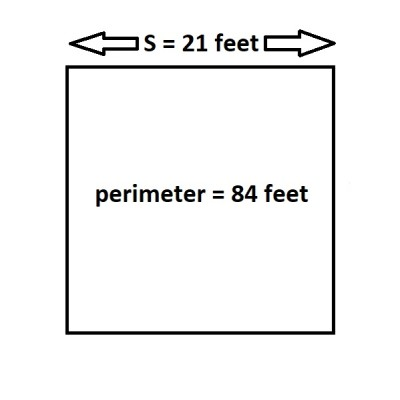A square with a perimeter of 84 square feet has a side length of 21 feet.

### What Does The Perimeter Of A Square Mean?

The perimeter of a square basically tells us the distance around the outside edge of the square.  In other words, how far would you have to walk to go around the square.

### Can The Perimeter & Area Of A Square Be The Same?

The perimeter and area of a square can have the same numerical value in a certain case.  However, the units are always different, since:

• Perimeter has linear units (feet, inches, meters, etc.)
• Area has square units (square feet, square inches, square meters, etc.)

Let’s use the formula for perimeter and area of a square to find out when they have the same value.

Remember that for a square with a side length of S:

• Perimeter = P = 4S
• Area = A = S2

So, the perimeter and area are the same when:

• Perimeter = Area
• 4S = S2
• 0 = S2 – 4S
• 0 = S(S – 4)
• S = 0 or S = 4

So, there are two possible cases when the perimeter and area of a square have the same value:

• S = 0 (a trivial square with no area and zero side lengths and perimeter)
• S = 4 (a square with side lengths 4 units)

So, a square with a side length of 4 feet would have a perimeter of 4*4 = 16 feet and an area of 42 = 16 square feet.

## Conclusion

Now you know how to find the perimeter of a square in various cases.  You also know the answers to some common questions about squares and their perimeters.

You can learn about the uses of perimeter here.

You can learn how to find the area of a square here.

I hope you found this article helpful.  If so, please share it with someone who can use the information.